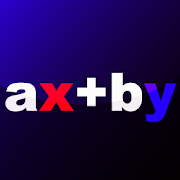# Bézout's Identity Calculation TrainingEveryone
An integer equation of the form ax + by = c is called "Bézout's identity".
Bézout's identity does not have enough conditional expressions for the number of variables. These are called "indefinite equations".
"Indefinite equations" have an infinite number of answers, but finding one of them requires a lot of computing power.

So this app will train you to find one answer!
If you try it, you'll notice that your brain gets a good workout.
By the way, indefinite equations may not have integer solutions, but this app only asks questions with integer solutions.

【Rules】
The question is an indefinite equation.
The moment you find one integer solution, it is treated as the correct answer, and the next question will be asked.
You have to compete in the time it takes to get six correct answers.
If you don't know the answer, you can change the question.
However, each time you change a question twice, the number of correct answers you have to get is increased by one.
Collapse

Review Policy

## What's New

You can now see the top three records and the record in the middle!
Collapse

Updated
September 18, 2020
Size
7.0M
Installs
10+
Current Version
1.1.0
Requires Android
4.1 and up
Content Rating
Everyone
Permissions
Offered By
Oka Ryunoske
Developer